# Year 4 Summer Block 5 Properties of shape

• Slides: 11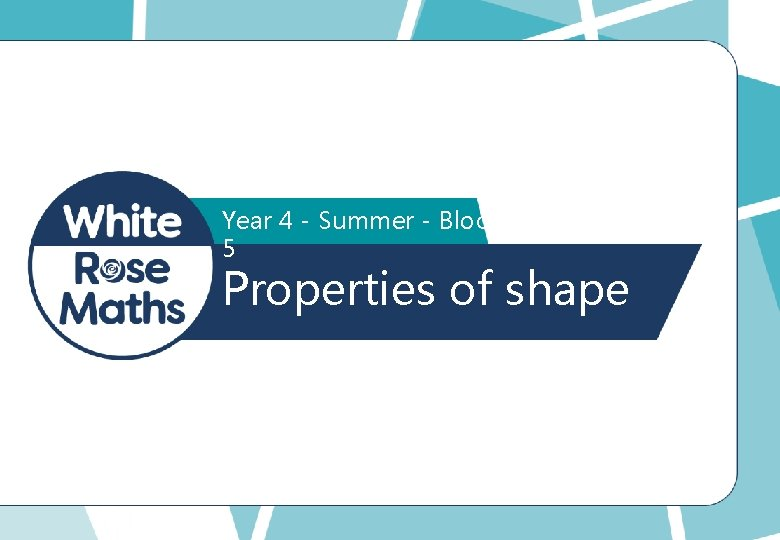Year 4 - Summer - Block 5 Properties of shapeI know the angle is not obtuse. Alex I know the angle is acute. I think the angle is roughly 45˚ Who is correct? Explain your reasons. 2 Teddy Whitney © White Rose Maths 2019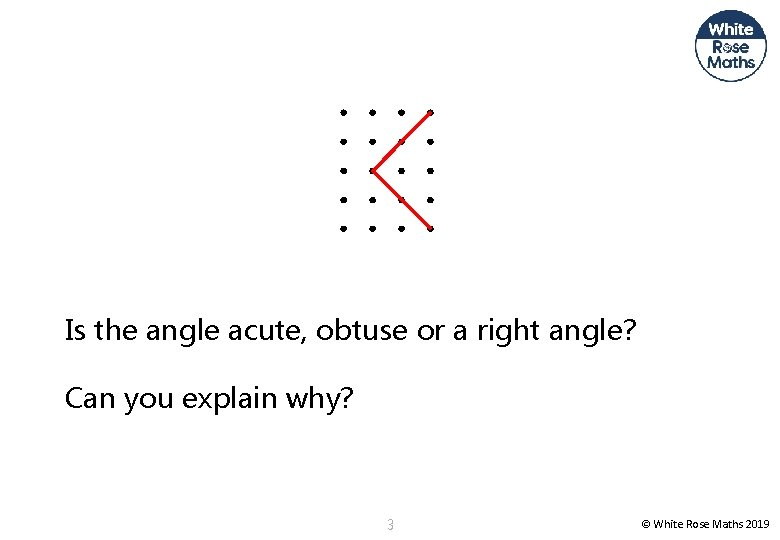Is the angle acute, obtuse or a right angle? Can you explain why? 3 © White Rose Maths 2019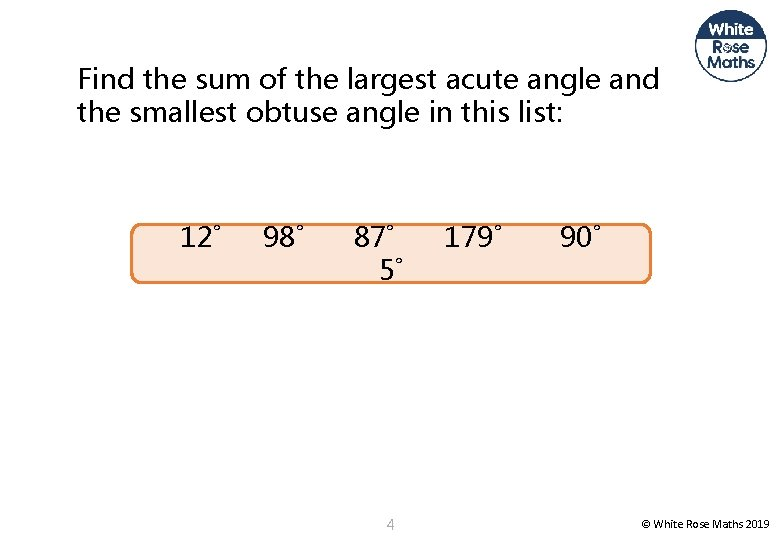Find the sum of the largest acute angle and the smallest obtuse angle in this list: 12˚ 98˚ 87˚ 5˚ 4 179˚ 90˚ © White Rose Maths 2019Angle A Angle B is bigger than Angle A because it has longer sides. Ro n Do you agree with Ron? Explain your thinking. 5 © White Rose Maths 2019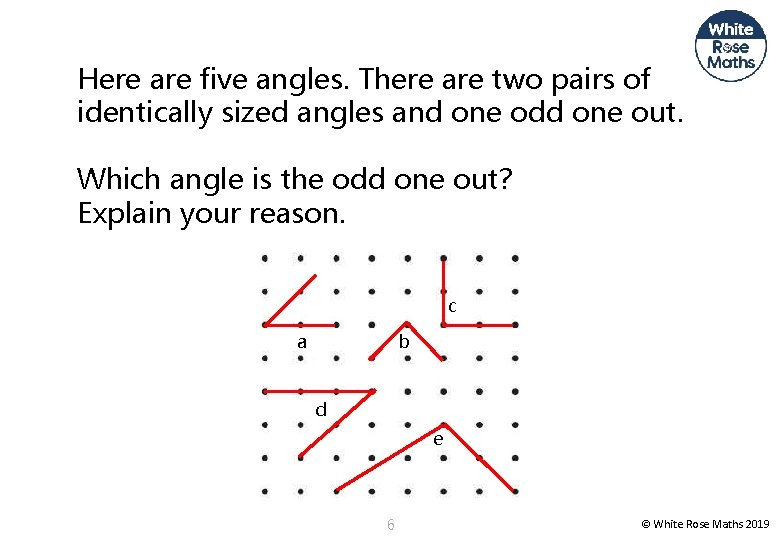Here are five angles. There are two pairs of identically sized angles and one odd one out. Which angle is the odd one out? Explain your reason. c a b d e 6 © White Rose Maths 2019I know the angle is not obtuse. Alex I know the angle is acute. I think the angle is roughly 45˚ Teddy Whitney Who is correct? Explain your reasons. All are correct. You may reason about how Whitney has come to her answer and discuss that the angle is about half a right angle. Half of 90 © White Rose Maths 2019 7 degrees is 45 degrees.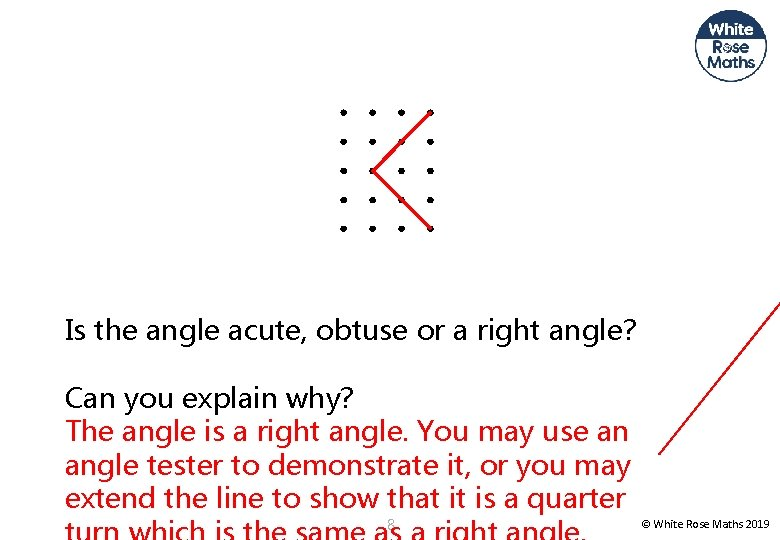Is the angle acute, obtuse or a right angle? Can you explain why? The angle is a right angle. You may use an angle tester to demonstrate it, or you may extend the line to show that it is a quarter 8 © White Rose Maths 2019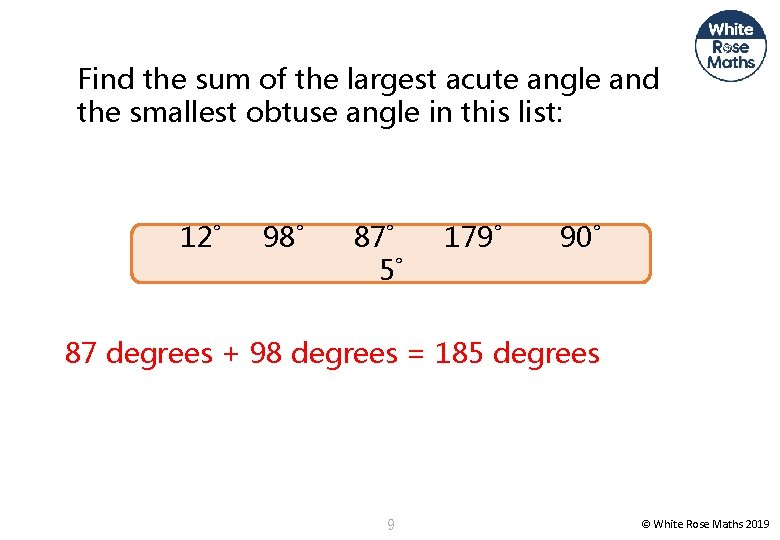Find the sum of the largest acute angle and the smallest obtuse angle in this list: 12˚ 98˚ 87˚ 5˚ 179˚ 90˚ 87 degrees + 98 degrees = 185 degrees 9 © White Rose Maths 2019Angle A Angle B is bigger than Angle A because it has longer sides. Ro n Do you agree with Ron? Explain your thinking. Angle A and angle B are the same size. Ron has mixed 10 © White Rose Maths 2019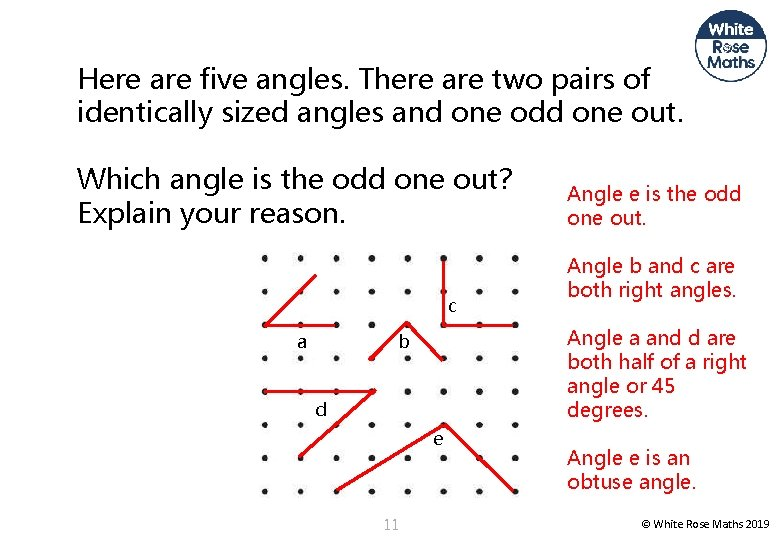Here are five angles. There are two pairs of identically sized angles and one odd one out. Which angle is the odd one out? Explain your reason. c a Angle b and c are both right angles. Angle a and d are both half of a right angle or 45 degrees. b d e 11 Angle e is the odd one out. Angle e is an obtuse angle. © White Rose Maths 2019